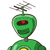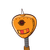# Use Euclid’s division lemma to show that the cube. of any positive integer is of the form 9m,9m+1 or 9m+8​

Use Euclid’s division lemma to show that the cube. of any positive integer is of the form 9m,9m+1 or 9m+8​

### 2 thoughts on “Use Euclid’s division lemma to show that the cube. of any positive integer is of the form 9m,9m+1 or 9m+8​”

1.## ★Important formula★

• (a+b)³= a³+3a²b+3ab²+b³
2.Let x be any positive integer and y = 3.

By Euclid’s division algorithm, then,

x = 3q+r, where q≥0 and r = 0, 1, 2, as r ≥ 0 and r < 3.

Therefore, putting the value of r, we get,

x = 3q

or

x = 3q + 1

or

x = 3q + 2

Now, by taking the cube of all the three above expressions, we get,

Case (i): When r = 0, then,

x2= (3q)3 = 27q3= 9(3q3)= 9m; where m = 3q3

Case (ii): When r = 1, then,

x3 = (3q+1)3 = (3q)3 +13+3×3q×1(3q+1) = 27q3+1+27q2+9q

Taking 9 as common factor, we get,

x3 = 9(3q3+3q2+q)+1

Putting = m, we get,

Putting (3q3+3q2+q) = m, we get ,

x3 = 9m+1

Case (iii): When r = 2, then,

x3 = (3q+2)3= (3q)3+23+3×3q×2(3q+2) = 27q3+54q2+36q+8

Taking 9 as common factor, we get,

x3=9(3q3+6q2+4q)+8

Putting (3q3+6q2+4q) = m, we get ,

x3 = 9m+8

Therefore, from all the three cases explained above, it is proved that the cube of any positive integer is of the form 9m, 9m + 1 or 9m + 8.